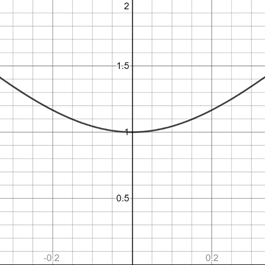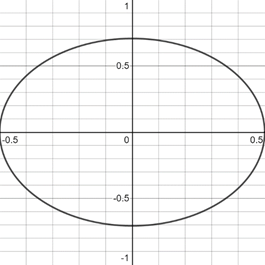# To graph : The equation y 2 − 9 x 2 = 1 by solve for y and the graphing the two equation corresponding to the positive and negative square roots.### Precalculus: Mathematics for Calcu...

6th Edition
Stewart + 5 others
Publisher: Cengage Learning
ISBN: 9780840068071### Precalculus: Mathematics for Calcu...

6th Edition
Stewart + 5 others
Publisher: Cengage Learning
ISBN: 9780840068071

#### Solutions

Chapter 1.9, Problem 30E
To determine

## To graph: The equation y2−9x2=1 by solve for y and the graphing the two equation corresponding to the positive and negative square roots.

Expert Solution

### Explanation of Solution

Given information:

The equation of hyperbola y29x2=1 .

Graph:

The graph of hyperbola y29x2=1 by solving for y .

Adding 9x2 in the equation of hyperbola,

9x29x2+y2=1+9x2y2=1+9x2

Take under root on both side of the equation,

y=±1+9x2

From the two equations graph of hyperbola is described:

y=+1+9x2,y=1+9x2

The graph of the equation y=+1+9x2 represents upper half of the hyperbola because y is always greater than or equal to 0.

1+9x201+9x2019x2±13x

So, the values of x is greater than or equal to ±13 .

Thus, the graph appropriate in the [13,13]×[0,2] viewing rectangle

The graph of the equation y=1+9x2 can be sketched using the table,

 x y 0 1 13 2 −13 2

The graph of equation is provided below,The graph of the equation y=1+9x2 represents lower half of the hyperbola because y is always less than or equal to 0.

1+9x201+9x2019x2±13x

So, the values of x is less than or equal to ±13 .

Thus, the graph appropriate in the [13,13]×[-1,0] viewing rectangle

The graph of the equation y=1+9x2 can be sketched using the table,

 x y 0 −1 13 −2 −13 −2

The graph of equation is provided below,the graph of circle 4x2+2y2=1 appropriate in the [12,12]×[-1,1] viewing rectangle

The graph of both equations in same figure is provided below,Interpretation:

The graph of an equation in a viewing screen is a viewing rectangle.

The x -values to range from a minimum value of xmin =a to a maximum value of xmax =b

The y -values to range from minimum value of ymin =c to a maximum value of ymax =d

Then, the display portion of the graph lies in the rectangle [a,b]×[c,d]={(x,y)|axb,cyd}

### Have a homework question?

Subscribe to bartleby learn! Ask subject matter experts 30 homework questions each month. Plus, you’ll have access to millions of step-by-step textbook answers!